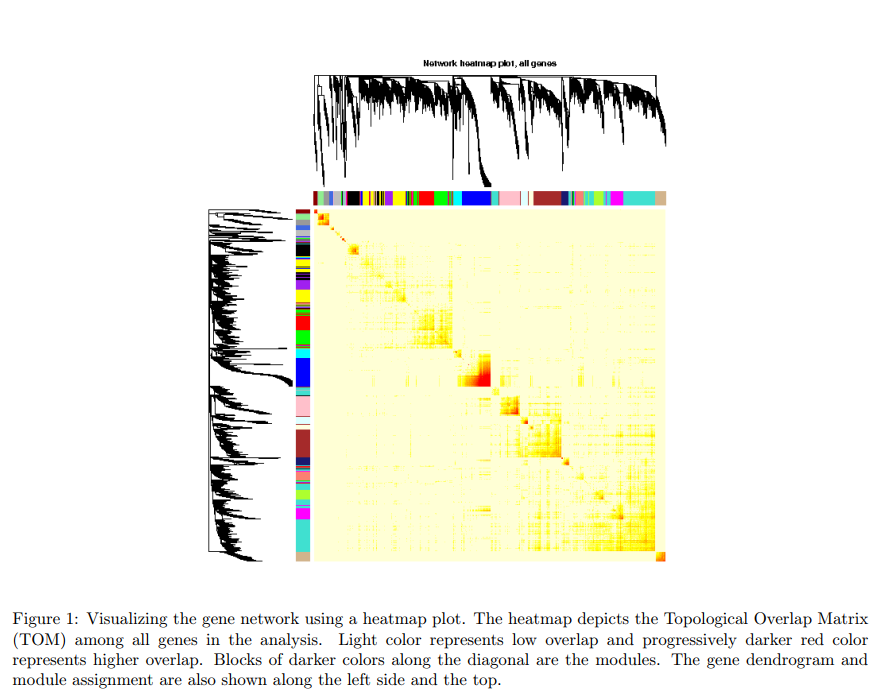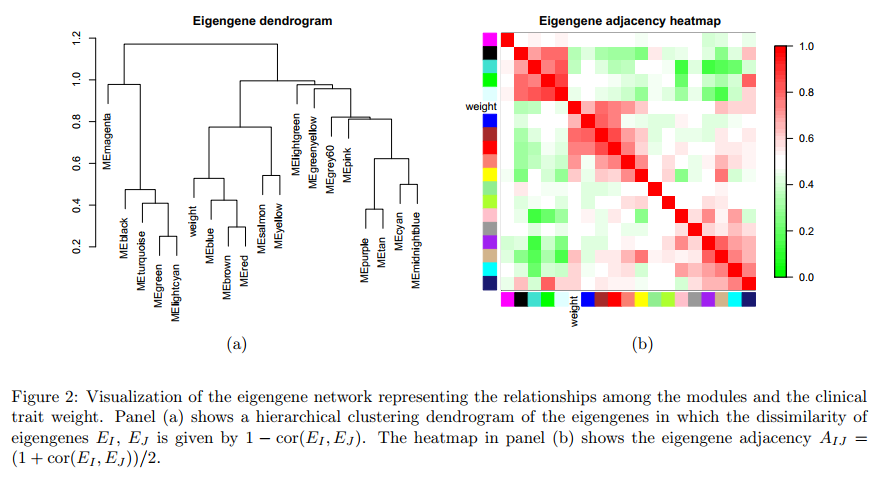# 5. 网络可视化

## 5.1. 导入处理的数据：

``````# 设定工作目录
setwd("/home/cxt/data/R/")
# 导入包
library(WGCNA)
# 设置将strings不要转成factors
options(stringsAsFactors=F)

# 允许使用最大线程
# 或者直接指定线程数

# 导入表达与临床数据
lnames
# 导入共表达网络及模块
lnames
nGenes = ncol(datExpr)
nSamples = nrow(datExpr)
``````

## 5.2. Gene Network可视化

``````# 重新计算拓扑重叠:通过保存TOM可以更有效地实现这一点
# 重复模块检过程
dissTOM = 1-TOMsimilarityFromExpr(datExpr, power = sft\$powerEstimate);
# 使用powe转换dissTOM，使中等强度的连接在heatmap中更加可见
plotTOM = dissTOM^sft\$powerEstimate;
# 将对角线设为NA，这样画的更好
diag(plotTOM) = NA;
# 调用绘图函数
sizeGrWindow(9,9)
TOMplot(plotTOM, geneTree, moduleColors, main = "Network heatmap plot, all genes")
``````

``````nSelect = 400
# 为了重现性，我们设置随机种子
set.seed(10);
select = sample(nGenes, size = nSelect);
selectTOM = dissTOM[select, select];
# 没有一种简单的方法可以将聚类树限制为基因的子集，因此我们必须重新聚类。
selectTree = hclust(as.dist(selectTOM), method = "average")
selectColors = moduleColors[select];
# 打开绘图窗口
sizeGrWindow(9,9)
# 将dissimilarity转化为power，比如10，通过有效地改变，可以让情节更加丰富
# 调色板;将对角线设置为NA也可以提高剧情的清晰度
plotDiss = selectTOM^sft\$powerEstimate;
diag(plotDiss) = NA;
TOMplot(plotDiss, selectTree, selectColors, main = "Network heatmap plot, selected genes")
``````## 5.3. Eigengenes Network可视化

``````# 重新计算module eigengenes
MEs = moduleEigengenes(datExpr, moduleColors)\$eigengenes
# 从临床特征中分离出体重
weight = as.data.frame(datTraits\$weight_g);
names(weight) = "weight"
# 将体重添加到现有的module eigengenes
MET = orderMEs(cbind(MEs, weight))
# 绘制特征基因与临床特征（体重）之间的关系
sizeGrWindow(5,7.5);
par(cex = 0.9)
plotEigengeneNetworks(MET, "", marDendro = c(0,4,1,2), marHeatmap = c(3,4,1,2), cex.lab = 0.8, xLabelsAngle
= 90)
``````

``````# 绘制聚类提
sizeGrWindow(6,6);
par(cex = 1.0)
plotEigengeneNetworks(MET, "Eigengene dendrogram", marDendro = c(0,4,2,0),
plotHeatmaps = FALSE)
# 绘制热图矩阵(注意:此图将覆盖聚类树)
par(cex = 1.0)
plotEigengeneNetworks(MET, "Eigengene adjacency heatmap", marHeatmap = c(3,4,2,2),
plotDendrograms = FALSE, xLabelsAngle = 90)

``````### 评论

###### Your browser is out of date!

Update your browser to view this website correctly. Update my browser now

×